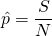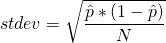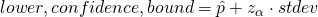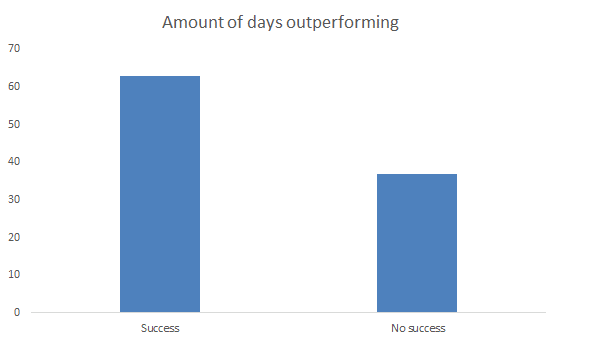The assessment of the predictability of a (day) trading system is essential in designing a successful trading rule. This is not only because higher success rates lead to higher portfolio returns. Also the decrease the variance of the performance is valuable. This can be very valuable in highly volatile markets.

## Day trading success rate formula

The assessment of significant predicitability can be done by using a normal approximation of the binomial distribution to assess significant difference between the proportions of 2 categories. First, you need to define the success rate. This is simply the amount of successes registered, S, divided by the total amount of recordings N:Secondly, the standard deviation of the proportion can be estimated based on the following formula:Since we are only interested in whether the system is better than a rondom 50% prediction 1-sided confidence intervals are used. The 1-sided confidence interval is calculated by using the observed success rate, the estimated standard deviation and the z-score on a certain significance level α.## Portfolio success rate example

Consider the following day trading system which tries to beat the market. Over the past 100 days, the trading system realized a higher return than the market in 63 out of 100 cases. In 37 cases, the return was lower. The million dollar question which can be asked is: is this trading system based on the recorded data able to predict the direction better than randomly going long or short. In other words, is the probability of success, 63% significantly larger than 50%?## Portfolio success rate interpretation

Using these confidence intervals, it is possible to know whether the success rate of a portfolio is significantly higher than 50%. In other words, it is possible to assess whether a trading system has significant predictive ability. This is simply done by assessing whether 50% lies in the range of the confidence interval for a given significance level. If it is not, than this means that a trading system has predictive ability.

## Summary

Assessing the predictability of a trading system is essential in the implementation of a sound trading strategy. This can be assessed by using a normal approximation of the binomial distribution to assess difference in proportions between to categories. Trading systems with higher predictability can have both higher and less volatile returns.